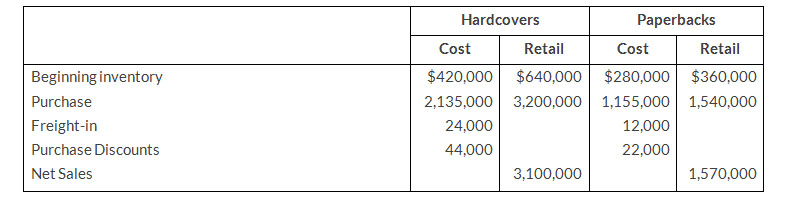# Inventories

Rayre Books uses the retail inventory method to estimate its monthly ending inventories. The following information is available for two of its departments of October 31, 2019.At Decemer31, Rayre Books takes a physical inventory at retail. The actual retail values of the inventories in each department are Hardcovers \$744,000 and Paperbacks \$335,000.

### Instructions

1. Determine the estimated cost of the ending inventory for each department at October 31, 2019, using the retail inventory method.
2. Compute the ending inventory at cost for each department at December 31, assuming the cost-to-retail ratios for the year are 75% for Hardcovers and 75% for Paperbacks.

### Solution

a.
Rayre Booksb.
Cost of ending inventory (Hardcovers) = \$744,00 x 65% = \$483,600
Cost of ending inventory (Paperbacks) = \$335,00 x 75% = \$251,250